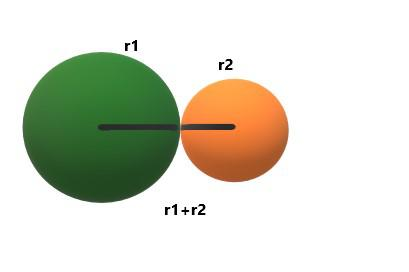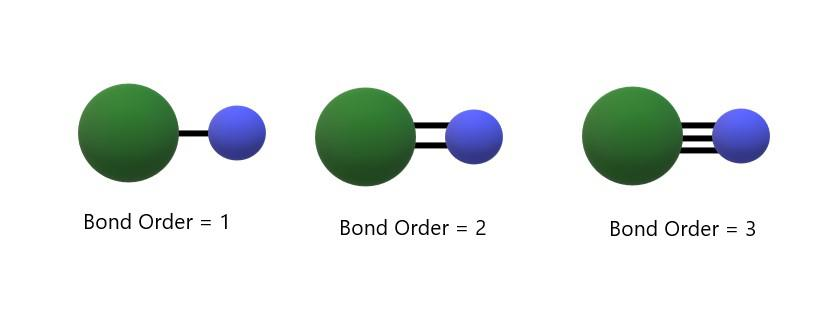Open in App
Not now

# Bond Order Formula

• Last Updated : 02 Jan, 2023

Chemistry deals with various particles, atoms, or elements and the chemical reactions between them. All the chemical reactions that take place are directly the result of the formation or breaking of bonds between the elements. Whenever two atoms combine to form a molecule, they form bonds between themselves. These bonds may be ionic or covalent. In both cases, the electrons are the ones that play an important role in bond formation as any bond is the result of the transfer or sharing of electrons between two atoms. Thus the number of electrons involved in the formation of a molecule decides the number of bonds between the atoms. This leads to the definition of the term Bond Order. The term bond order was first coined by Linus Pauling and he is known to introduce the concept of bond order. Bond order is a very crucial and significant term in molecular orbital theory (MOT). In this article, we shall learn what is bond order and how to calculate it.

## Bond Order

The bond order may be defined as the number of pairs of electrons that are present between the two atoms in a molecule or we can say that the number of bonds that are present between the two atoms defines the bond order of that molecule. Bond order is the result of the difference in bonding and antibonding molecular orbitals in a molecule.

## Properties of Bond Order

• The strength of a bond is measured with respect to its bond order. Higher the bond order, the more the strength of the bond between the atoms and more is the energy required to break the bond.
• The stability of a molecule is also measured by the bond order. Higher the bond order, the more stable the molecule.
• Bond order is determined by the electronic configuration of the atoms.
• Bond order is always a whole number except for the molecules that exhibit resonance bonding.
• Bond order cannot be zero.
• If the bond order comes out to be a fraction or zero, then no bonding takes place between the atoms.
• Bond order can never be negative.
• Bond order is also used to determine the bond length. Higher the bond order, the lower the bond length.
• Hybridization of an atom can also be known from its bond order.
• Concept of bond order is used in molecular dynamics and bond order potentials.

## Bond Length

The length of the bond that exists between the nuclei of two atoms is called bond length. It is a measure of the strength of the molecule.Bond Length

## Bond Order Formula

The bond order formula considers both the number of electrons in bonding and antibonding molecular orbitals of the molecule. It can be calculated by finding the difference between the number of electrons in bonding and antibonding molecular orbitals of the molecule and dividing the difference by 2. Thus the bond order formula can be written as:where,
Nb is the number of electrons in bonding molecular orbitals,
Na is the number of electrons in antibonding molecular orbitals

If the bond order is 1 then a single bond is formed between atoms, if BO is 2 then a double bond and a triple bond in case the bond order is 3.Also, Check

## Solved Examples Bond Order Formula

Example 1: Calculate the bond order of the O2 molecule.

Solution:

The electronic configuration of an O2 molecule according to the Molecular Orbital Theory is as follows:

(σ1s)21s)2(σ2s)22s)2(σ1pz​)2(π2px​)2 = (π2py​)22px​)1 = (π2py​)1

From the above electronic configuration,

Nb = 10, Na = 6

Thus,= 1/2 (10 -6) = 2

So, the bond order of the O2 molecule is 2 which means that a double bond exists between the two O atoms.

Example 2: Calculate the bond order of the N2 molecule.

Solution:

The electronic configuration of the N2 molecule according to the Molecular Orbital Theory is as follows:

(σ1s)21s)2(σ2s)22s)2(σ1pz​)2(π2px​)2 = (π2py​)2

From the above electronic configuration,

Nb = 10, Na = 4

Thus,= 1/2 (10 -4) = 3

So, the bond order of the N2 molecule is 3 which means that a triple bond exists between the two N atoms.

Example 3: Arrange the following in increasing order of their bond length: O22-, O2, O2, O2+

Solution:

Bond order of the given molecules is as follows:

O22-= 1
O2 = 2
O2= 2.5
O2+ = 1.5

As we know the higher the bond order, the lower the bond length. Thus the increasing order of the given molecules is as follows:

O22- < O2+ < O2 <O2

## FAQs on Bond Order Formula

Question 1: Define bond order and how can you calculate it?

Bond order may be defined as the number of pairs of electrons that are present between the two atoms in a molecule or we can say that the number of bonds that are present between the two atoms defines the bond order of that molecule. Bond order is the result of the difference in bonding and antibonding molecular orbitals in a molecule. It can be calculated using the bond order formula which is as follows:where,
Nb is the number of electrons in bonding molecular orbitals,
Na is the number of electrons in antibonding molecular orbitals.

Question 2: Why a shorter bond length leads to higher bond energy?

A shorter bond length has higher bond energy because if the bond length is small then the two atoms are closer to each other and the electrons that participate in the bond formation are more tightly held by the nucleus. Thus there are greater attraction forces when the atoms are close to each other or the bond length is small. Thus the bond energy increases in a small bond length.

Question 3: What are some methods that can be used to find bond length?

Bond length can be determined using various methods like spectroscopy, X-ray diffraction, and electron diffraction.

Question 4: Which hybridization state is shown in a single, double and triple bond?